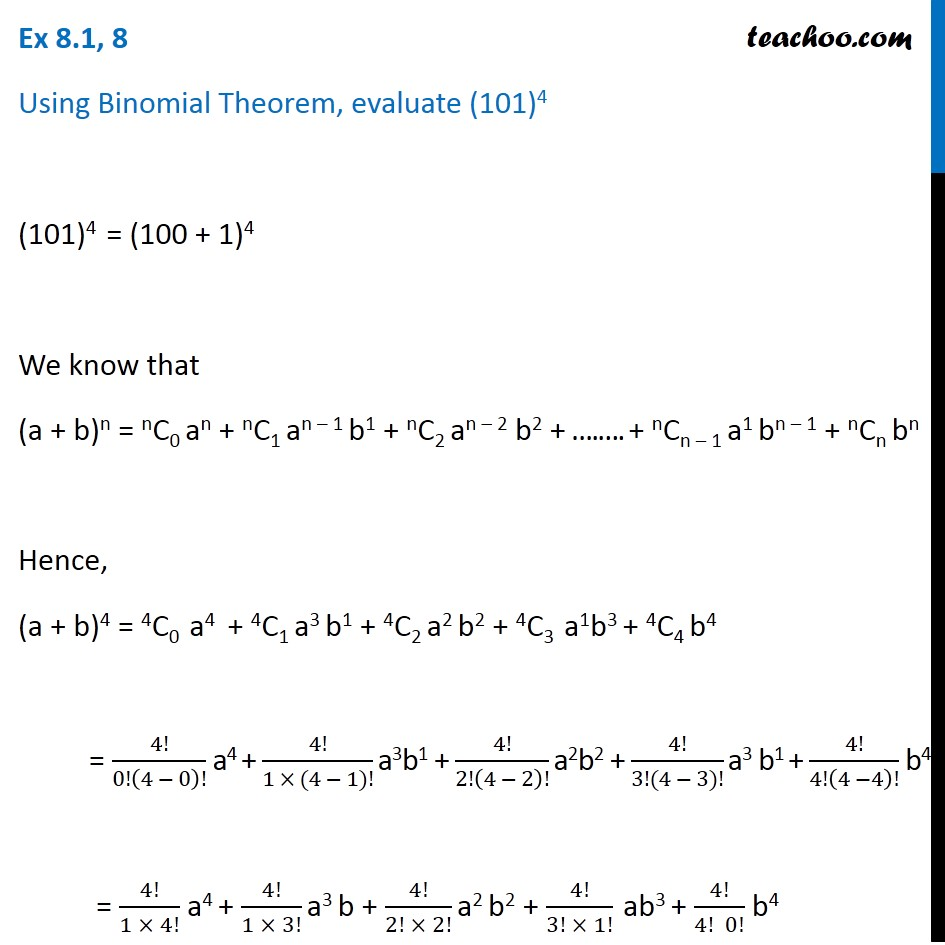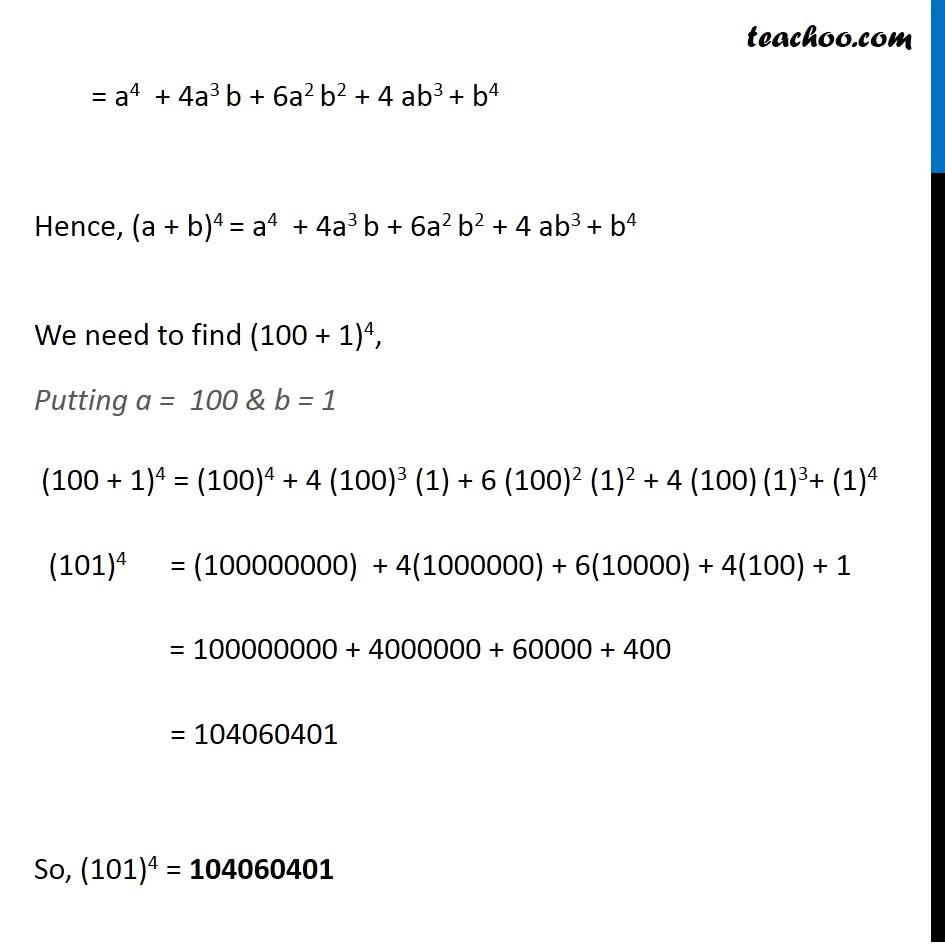Ex 7.1

Chapter 7 Class 11 Binomial Theorem
Serial order wiseLearn in your speed, with individual attention - Teachoo Maths 1-on-1 Class

### Transcript

Ex 7.1, 8 Using Binomial Theorem, evaluate (101)4 (101)4 = (100 + 1)4 We know that (a + b)n = nC0 an + nC1 an – 1 b1 + nC2 an – 2 b2 + ….…. + nCn – 1 a1 bn – 1 + nCn bn Hence, (a + b)4 = 4C0 a4 + 4C1 a3 b1 + 4C2 a2 b2 + 4C3 a1b3 + 4C4 b4 = 4!/0!(4 − 0)! a4 + 4!/(1 × (4 − 1)!) a3b1 + 4!/2!(4 − 2)! a2b2 + 4!/(3!(4 − 3)!) a3 b1 + 4!/4!(4 −4)! b4 = 4!/(1 × 4!) a4 + 4!/(1 × 3!) a3 b + 4!/(2! × 2!) a2 b2 + 4!/(3! × 1!) ab3 + 4!/(4! 0!) b4 = a4 + 4a3 b + 6a2 b2 + 4 ab3 + b4 Hence, (a + b)4 = a4 + 4a3 b + 6a2 b2 + 4 ab3 + b4 We need to find (100 + 1)4, Putting a = 100 & b = 1 (100 + 1)4 = (100)4 + 4 (100)3 (1) + 6 (100)2 (1)2 + 4 (100) (1)3+ (1)4 (101)4 = (100000000) + 4(1000000) + 6(10000) + 4(100) + 1 = 100000000 + 4000000 + 60000 + 400 = 104060401 So, (101)4 = 104060401